A pulley fixed to the ceilling carries a string with blocks of mass m and 3 m attached to its ends. The masses of string and pulley are negligible. When the system is released, its centre of mass moves with what acceleration

(1) 0

(2) g/4

(3) g/2

(4) –g/2

High Yielding Test Series + Question Bank - NEET 2020

Difficulty Level:

A solid sphere of mass 2 kg is resting inside a cube as shown in the figure. The cube is moving with a velocity $v=\left(5t\text{\hspace{0.17em}}\stackrel{^}{i}+2t\text{\hspace{0.17em}}\stackrel{^}{j}\right)m/s$. Here t is the time in second. All surface are smooth. The sphere is at rest with respect to the cube. What is the total force exerted by the sphere on the cube. (Take g = 10 m/s2)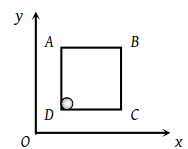1. $\sqrt{29}N$

2. 29 N

3. 26 N

4. $\sqrt{89}\text{\hspace{0.17em}}N$

Concept Questions :-

Types of forces
High Yielding Test Series + Question Bank - NEET 2020

Difficulty Level:

A block B is placed on block A. The mass of block B is less than the mass of block A. Friction exists between the blocks, whereas the ground on which the block A is placed is taken to be smooth. A horizontal force F, increasing linearly with time begins to act on B. The acceleration aA and aB of blocks A and B respectively are plotted against t. The correctly plotted graph is-

(1)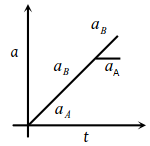(2)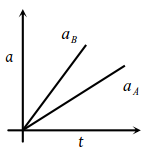(3)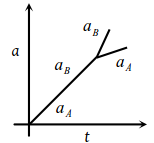(4)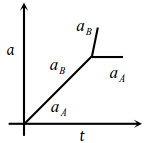Concept Questions :-

Friction
High Yielding Test Series + Question Bank - NEET 2020

Difficulty Level:

The force-time (Ft) curve of a particle executing linear motion is as shown in the figure. The momentum acquired by the particle in time interval from zero to 8 second will be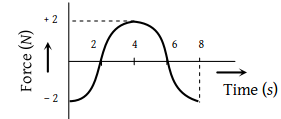(1) – 2 N-s

(2) + 4 N-s

(3) 6 N-s

(4) Zero

Concept Questions :-

Newton laws
High Yielding Test Series + Question Bank - NEET 2020

Difficulty Level:

A body of 2 kg has an initial speed 5ms–1. A force acts on it for some time in the direction of motion. The force time graph is shown in figure. The final speed of the body.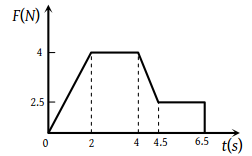(1) 9.25 ms–1

(2) 5 ms–1

(3) 14.25 ms–1

(4) 4.25 ms–1

Concept Questions :-

Newton laws
High Yielding Test Series + Question Bank - NEET 2020

Difficulty Level:

A particle of mass m, initially at rest, is acted upon by a variable force F for a brief interval of time T. It begins to move with a velocity u after the force stops acting. F is shown in the graph as a function of time. The curve is an ellipse.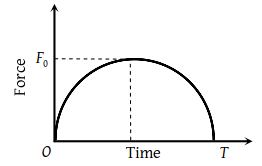(1) $u=\frac{\pi {F}_{0}^{2}}{2m}$

(2) $u=\frac{\pi {T}^{2}}{8m}$

(3) $u=\frac{\pi {F}_{0}T}{4m}$

(4) $u=\frac{{F}_{0}T}{2m}$

Concept Questions :-

Newton laws
High Yielding Test Series + Question Bank - NEET 2020

Difficulty Level:

A body of mass 3kg is acted on by a force which varies as shown in the graph below. The momentum acquired is given by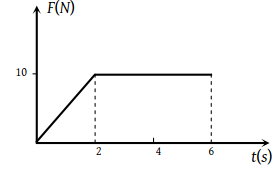(1) Zero

(2) 5 N-s

(3) 30 N-s

(4) 50 N-s

Concept Questions :-

Newton laws
High Yielding Test Series + Question Bank - NEET 2020

Difficulty Level:

The variation of momentum with time of one of the body in a two body collision is shown in fig. The instantaneous force is maximum corresponding to point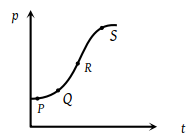(1) P

(2) Q

(3) R

(4) S

Concept Questions :-

Newton laws
High Yielding Test Series + Question Bank - NEET 2020

Difficulty Level:

Figures I, II, III and IV depict variation of force with time

 (I)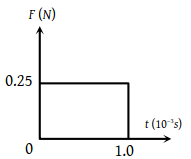(II)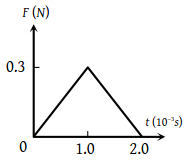(III)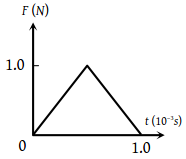(IV)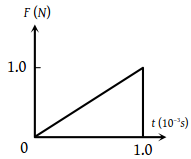The impulse is highest in the case of situations depicted. Figure

(1) I and II

(2) III and I

(3) III and IV

(4) IV only

Concept Questions :-

Newton laws
High Yielding Test Series + Question Bank - NEET 2020

Difficulty Level:

The masses of 10 kg and 20 kg respectively are connected by a massless spring as shown in figure. A force of 200 N acts on the 20 kg mass. At the instant shown, the 10 kg mass has acceleration 12 m/sec2. What is the acceleration of 20 kg mass?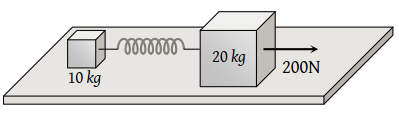(1) 12 m/sec2

(2) 4 m/sec2

(3) 10 m/sec2

(4) Zero

Concept Questions :-

Spring force